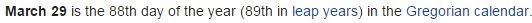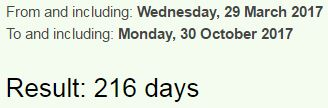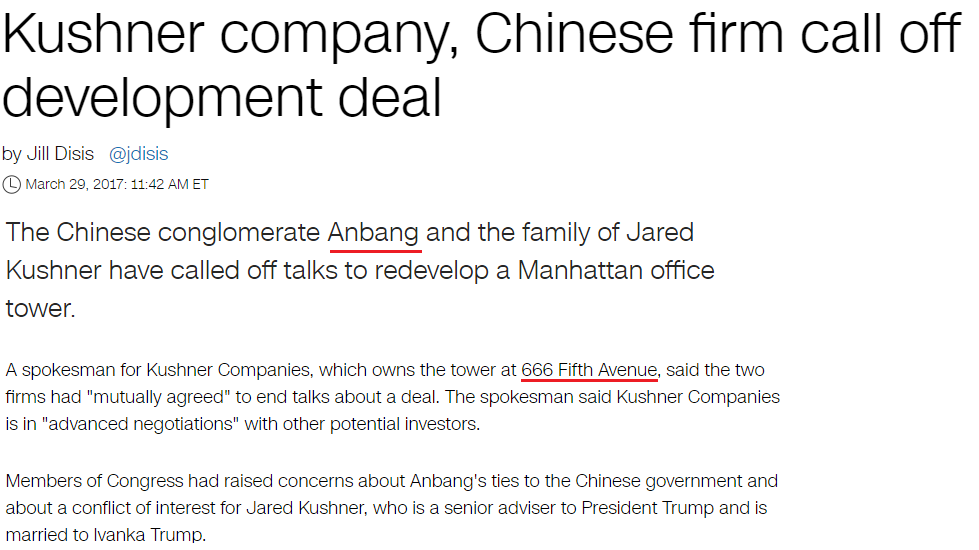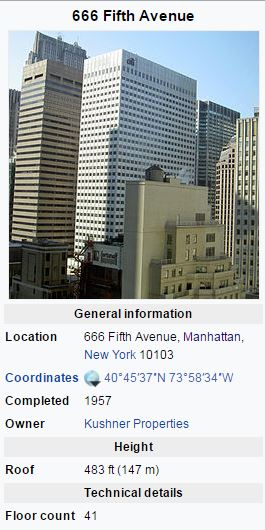CipherMar 30, 2017

Ivanka Trump is making her White House job official + Jared Kushner 666 Fifth avenueShe assumed office on 3/29/2017 which is exactly 151 days after her birthday
Ivanka Trump = 18+5+26+13+16+26 + 7+9+6+14+11 = 151 (Reverse Ordinal)Twenty ninth of March = 7+4+4+4+7+2 + 4+9+4+7+1 + 3+3 + 5+8+9+6+1 = 88 (Reverse Reduced)
Trump = 20+18+21+13+16 = 88 (Ordinal)It's also 216 days to her next birthday (6x6x6 = 216)She's born on 30th of October - Thirtieth of October = 20+8+9+18+20+9+5+20+8 + 15+6 + 15+3+20+15+2+5+18 = 216 (Ordinal)

http://edition.cnn.com/2017/03/29/politics/ivanka-trump-white-house-job/index.htmlIvanka Trump is making her White House job official = 9+4+1+5+2+1 + 2+9+3+4+7 + 9+1 + 4+1+2+9+5+7 + 8+5+9 + 5+8+9+2+5 + 8+6+3+1+5 + 1+6+2 + 6+6+6+9+3+9+1+3 = 211 (Reduced)
Ivanka Trump = 9+4+1+5+2+1 + 2+9+3+4+7 = 47 (Reduced)
Trump = 7+9+6+14+11 = 47 (Reverse Ordinal)She's known for being a boardroom judge on Trump's TV show "The Apprentice"
The Apprentice = 7+19+22 + 26+11+11+9+22+13+7+18+24+22 = 211 (Reverse Ordinal)
Judge = 10+21+4+7+5 = 47 (Ordinal)

She is "Assistant to the president"Thirty three = 7+19+18+9+7+2 + 7+19+9+22+22 = 141 (Reverse Ordinal)

On the day Ivanka took the white house job the media was talking about Jared Kushner -

It was a date with 42 numerology - 3/29/2017 - 3+29+2+0+1+7
"Kushner" = 2+3+10+8+5+5+9 = 42 (S Exception)"Anbang" = 8+4+7+8+4+2 = 33 (Reverse Reduced)
"Thirty three" = 2+8+9+9+2+7 + 2+8+9+5+5 = 66 (Reduced)
"Kushner company" = 2+3+1+8+5+5+9 + 3+6+4+7+1+5+7 = 66 (Reduced)
Ivanka married into Kushner family - "Kushner" = 2+3+1+8+5+5+9 = 33 (Reduced)Kushner properties = 2+3+1+8+5+5+9 + 7+9+6+7+5+9+2+9+5+1 = 93 (Reduced)
Kushner = 16+6+8+19+13+22+9 = 93 (Reverse Ordinal), Saturn = 93 (Ordinal)
Note that building is 147 meters tall (147 is 223 in octal system) both are very special numbers to Freemasonry

The building is 41 stories tall and this is coming exactly 41 weeks before Jared's next birthday (1/10/2018)